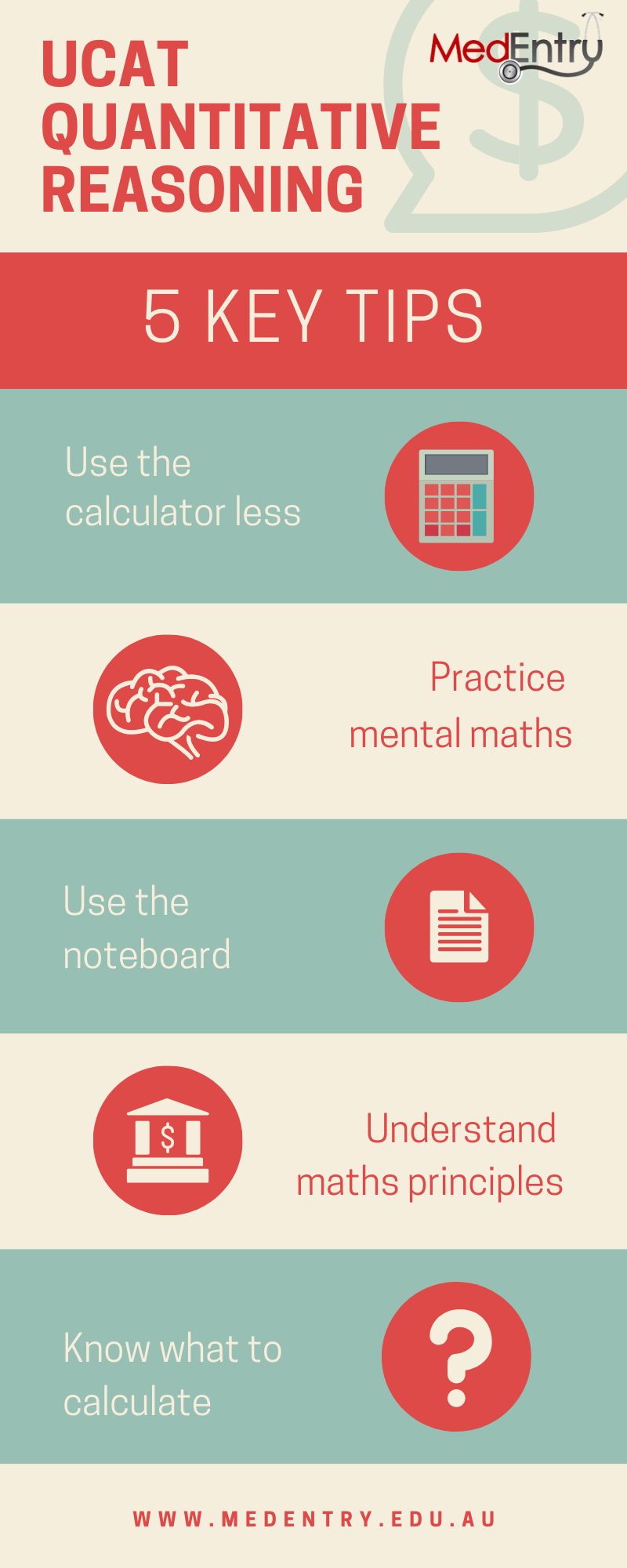## Top Tips for UCAT Quantitative Reasoning4 days ago by Robert

The Quantitative Reasoning subtest of UCAT can be challenging, even for those who are proficient at maths. This is largely due to the extreme time pressure placed on students in this UCAT subtest.

The commonest mistake made by students in the UCAT Quantitative Reasoning subtest is to approach it like you would approach a high school maths test – by calculating everything perfectly, checking your working and not being aware of common wrong answer traps.

To do well in the Quantitative Reasoning subtest of UCAT, you need a strategic approach. This blog outlines five key tips.

### Tip 1: Use the UCAT calculator lessBecause you are given a calculator in UCAT, many students feel the need to use it – either to ensure that their calculations are accurate, or to ‘check’ their calculations. However, the calculator significantly slows down the process of answering UCAT questions.

The highest performers in the Quantitative Reasoning subtest rely on the calculator less. They recognise that not all UCAT questions need to be answered by making a calculation (for example, some questions can be answered by estimation, ‘eyeballing’ the data or simply reading off the data provided).

Sometimes it will be necessary to use the UCAT calculator to arrive at the correct option. If this is the case, there are ways to ensure you use the UCAT calculator efficiently. One simple tip is to use the keypad on your keyboard instead of clicking the numbers with your mouse.

MedEntry's Calculator Keypad trainer will build up your muscle memory in performing various calculator operations, enabling you to answer UCAT Quantitative Reasoning questions quickly and accurately.

### Tip 2: Practice mental maths in the lead up to UCAT

Using your mental maths skills (that is, making simple calculations in your head) is far more efficient and time-saving than using the UCAT calculator. However, many students are rusty with simple operations such as addition, subtraction, multiplication and division.

Practice using your mental maths skills on a daily basis to get yourself ready for UCAT. Instead of reaching for a calculator whenever you have to make a calculation, try to do it in your head. MedEntry's Arithmetic Trainer will help you to develop these skills under timed conditions.

In the UCAT Quantitative Reasoning subtest, although some questions may seem like they require a complicated calculation, most can be answered relatively quickly – for example, by estimating, rounding up or down, or by using a simple shortcut.

### Tip 3: Use your UCAT noteboard

Many simple mistakes in the Quantitative Reasoning subtest are made due to careless errors in calculation. In fact, UCAT question writers commonly include incorrect answer options which ‘trap’ students who make such errors.

The UCAT places enormous stress on your short term memory, as you are required to interpret and manipulate the information provided. Reduce the strain on your brain by using your UCAT noteboard. In fact, of all subtests, the noteboard is probably most useful in the Quantitative Reasoning section (as well as UCAT Decision Making).

Write down important numbers, and important calculations to avoid making careless errors.

### Tip 4: Understand important mathematical principles

The UCAT Quantitative Reasoning subtest covers similar topics every year. Ensure you are familiar with the following concepts:

• Ratios, percentages and fractions
• Percentage change
• Speed, distance and time
• Geometry (particularly areas and volumes)
• Mean, median and mode
• Converting between units

To do well in the Quantitative Reasoning subtest, it is also important that you are comfortable with interpreting data from graphs, tables and other diagrams. MedEntry's Graph/Table trainer will help you develop this skill quickly.

### Tip 5: Understand what to calculate

When you are first presented with a Quantitative Reasoning UCAT question, it is easy to launch into making calculations without recognising what it actually is that you have to calculate. Remember that the Quantitative Reasoning subtest is not a maths test – it is a reasoning test that involves mathematics.

Therefore, before launching into answering a UCAT question, take a few moments to consider what you actually need to calculate to arrive at the answer. Write it down on your UCAT noteboard if necessary. If you make an incorrect calculation, it is likely that a wrong answer trap will be waiting for you.

With the right preparation and approach, you can excel in UCAT Quantitative Reasoning!